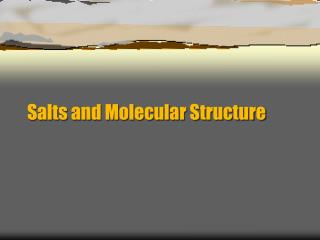DownloadDownload PresentationSalts and Molecular Structure

# Salts and Molecular Structure

Télécharger la présentation## Salts and Molecular Structure

- - - - - - - - - - - - - - - - - - - - - - - - - - - E N D - - - - - - - - - - - - - - - - - - - - - - - - - - -
##### Presentation Transcript

1. Salts and Molecular Structure

2. Hydrated metal ions • Charged metals ions also produce an acidic solution. • The metal itself does not act as a Brønsted-Lowry acid, but instead forms a hydrate that acts as a Brønsted-Lowry acid. • Typically the higher the charge on the metal ion, stronger the acidity of the hydrated ion. • Cu2+ + 5 H2O  Cu(H2O)52+ • Cu(H2O)52++ 5 H2O  CuOH(H2O)42+ + H+

3. Acidity of hydrated metal ions • M = metal • For Mn+, a small, highly charged ion, • M(H2O)xn++ H2O ⇌M(H2O)x-1OH(n-1)+ H3O+ • Calculate the pH of a 0.010 M AlCl3 solution. The Ka value for Al(H2O)63+ is 1.4 x 10-5.

4. Salts of weak acids and bases • For salts from a weak acid and weak base, the Ka and Kb of the ions must be compared. • If Ka> Kbthe solution will be acidic • If Ka< Kb the solution will be basic • If Ka= Kb the solution will be neutral

5. Problem • Predict whether an aqueous solutions of each of the following salts will be acidic, basic, or neutral. • NH4 C2H3O2 • NH4CN • Al2(SO4)3 • NH4F • Ka Al(H2O)63+ =1.4 x 10-5 • Ka HF = 7.2 x 10-4 • Ka HSO4- = 1.2x10-2 • Kb C2H3O2- = 5.6x10-10 • Kb CN- = 1.6x10-5 • Kb NH3 = 1.8 x 10-5

6. Molecular Trends in Acid Strength

7. Trends in Binary Nonmetal Hydride Activity • This applies to nonmetals only • From left to right, electronegativity increases, polarity increases.H+ removal is easier, and acidity increases. • From top to bottom, bond length increases, bond strength decreases, • H+ removal is easier, and acidity increases.

8. Halogen Strong Acids • All halogens are strong acids except fluorine. • All halogens have a high electronegativity. The short bond length of fluorine makes is a weak acid.

9. Oxyacids • With the same number of oxygen atoms around a central nonmetal (E),acid strength increases with the electronegativity of E. • HClO > HBrO > HIO •  With different numbers of oxygen atoms around E, acid strength increases with the number of oxygen atoms. • This is because oxygen atoms pull the electron density away from E, and H+ ismore easily removed.

10. Strong oxyanion acids • If there are at least 2 more oxygen atoms than hydrogen atoms, the acid will be strong. • H2SO4, HNO3, HClO3, HClO4

11. Leveling Effect • It is difficult to differentiate the strengths of strong acids or bases because of water. • Water can act like an acid or a base. • By LeChâtelier’s principle, the stronger the acid or base, the more water will shift to neutralize it. • Weak acids or bases are limited by their conjugate bases or acids, strong acids and base are limited by water.

12. Leveling effect • Water “levels” all strong acids (or bases) to an equal strength (HCl, HBr, and HI). • To determine the strength, Ka, of strong acids you have to dissolve it in an acid (weaker base than water), such as acetic acid, where different levels of protonation can occur.

13. Acidic Oxides (Acid Anhydrides) • Nonmetal oxides • O-X bond is strong and covalent. SO2, NO2, CO2 • When H-O-X grouping is dissolved in water, the O-X bond will remain intact. It will be the polar and relatively weak H-O bond that will tend to break, releasing a proton. • SO2 + H2O  H2SO3 • H2SO3 + H2O ⇌ H+ + HSO3-

14. Basic Oxides (Basic Anhydrides) • For metal oxides • O-X bond is ionic. K2O, CaO • If X has a very low electronegativity, the O-X bond will be ionic and subject to being broken in polar water, producing a basic solution. • K2 O+ H2O  KOH

15. Lewis Acids and Bases • This is the broadest definition. • An acid is an electron pair acceptor. • A base is an electron pair donor. • This is useful for: electron deficient compounds, polar double bonded compounds, metal ions, and protons (H­­­­+).

16. Lewis Acids and Bases • Lewis acid: electron pair acceptor • Lewis base: electron pair donor Lewis acid Lewis base

17. Acid base models

18. Acid-base Equilbria Chapter 15

19. Common Ion • A common ion is one that is both dissolved in an aqueous solution and introduced to the existing equilibrium system. • The shift in equilibrium as a result of the introduction of a common ion is called the common ion effect. • This is an application of Le Châtelier’sPrinciple.

20. Acidic Solutions Containing Common Ions. • The equilibrium concentration of H3O+ in a 1.0 M HF solution is 2.7 x 10-2 M, and the percent dissociation of HF is 2.7%. • Calculate [H3O+ ] and the percent dissociation of HF in a solution containing 1.0 M HF (Ka = 7.2 x 10-4) and 1.0 M NaF. *assume NaF dissociates at 100%